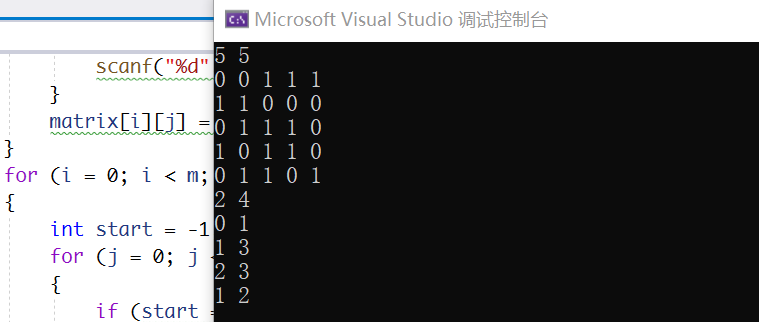2022-01-15 09:56

查找一个只包含0和1的矩阵中每行最长的连续1序列。 输入说明 输入第一行为两个整数m和n(0<=m,n<=100)表示二维数组行数和列数，其后为m行数据，每行n个整数（0或1），

• 写回答
• 好问题 提建议
• 追加酬金
• 关注问题
• 收藏
• 邀请回答

1条回答默认 最新

•LYSnowy 2022-01-15 10:33
已采纳

#include <stdio.h>
#include <malloc.h>

int main()
{
int m, n;
scanf("%d %d", &m, &n);

int i, j;
int** matrix = (int**)malloc(sizeof(int*) * m);
for (i = 0; i < m; ++i)
{
matrix[i] = (int*)malloc(sizeof(int) * (n+1));
}

for (i = 0; i < m; i++)
{
for (j = 0; j < n; j++)
{
scanf("%d", &matrix[i][j]);
}
matrix[i][j] = 0;
}
for (i = 0; i < m; i++)
{
int start = -1, end = -1, max = 0, res_x = -1, res_y = -1;
for (j = 0; j < n + 1; j++)
{
if (start == -1 && matrix[i][j] == 1)
{
start = j;
end = j;
}
if (start != -1 && matrix[i][j] == 1)
{
end = j;
}
if (start != -1 && matrix[i][j] == 0)
{
if (end - start + 1 > max)
{
max = end - start + 1;
res_x = start;
res_y = end;
}
start = -1;
end = -1;
}
}
printf("%d %d\n", res_x, res_y);
}
}评论
解决 无用
打赏 举报